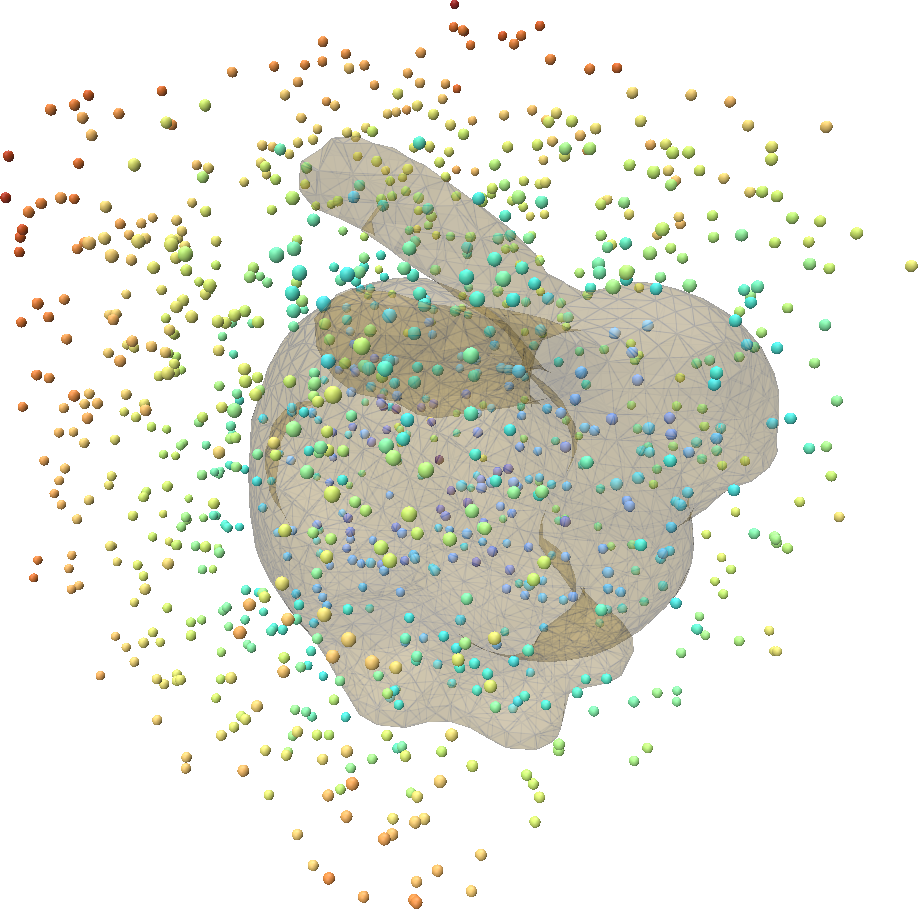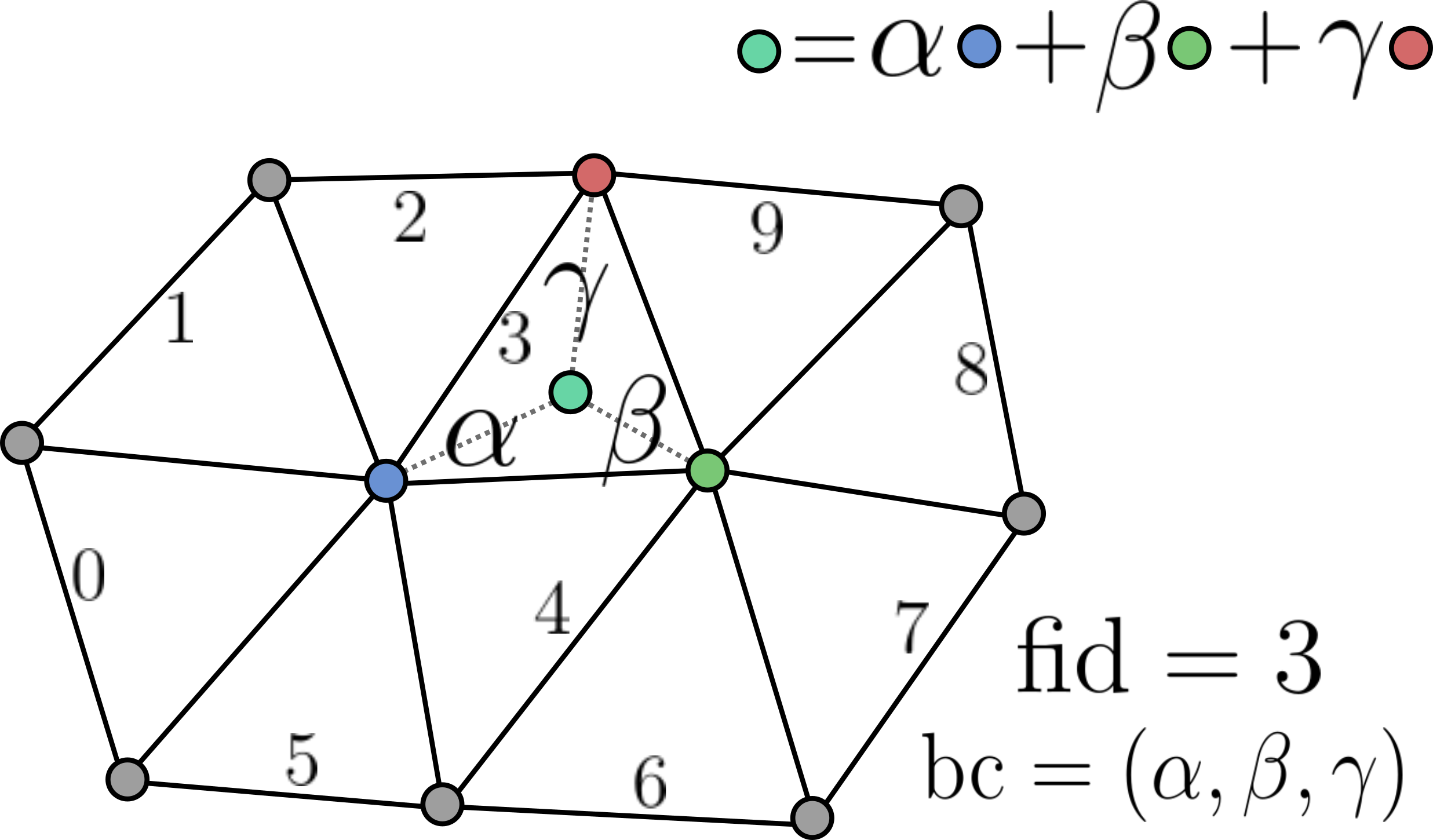# Computing Signed Distances (SDFs) to Meshes

Many applications require a signed distance function (SDF) representation for a 3D shape. For example, many shape reconstruction neural networks such as DeepSDF require such a representation for training. Unfortunately, most 3D shape data is stored as a triangle mesh, making SDFs not readily available. Point-Cloud-Utils makes it very easy to extract signed distances from a triangle mesh.

To extract an SDF for a triangle mesh, the mesh must be of relatively good quality (manifold, watertight, no sliver triangles, etc...). To clean up a mesh for SDF extraction see Making a triangle mesh watertight.

## SDF Overview

A signed distance function $$f : \mathbb{R}^3 \rightarrow \mathbb{R}$$ maps 3D points $$x \in \mathbb{R}^3$$ to the nearest distance between $$x$$ and some surface $$S$$. In other words: $$f(x) = \min_{x' \in S} |x - x'|_2$$ The zero level set of the SDF $$f$$ are precisely the set of points which lie on the surface. i.e. $$S = {x : f(x) = 0}$$ The image below shows a plot of the SDF for the boundary of the letters PCU.## Computing an SDF to a Mesh

We can compute the signed distance of a set of points in Point-Cloud-Utils in the following way:

import numpy as np
import point_cloud_utils as pcu

# 1000 random query points to compute the SDF at
query_pts = np.random.rand(1000, 3)

# sdf is the signed distance for each query point
# fid is the nearest face to each query point on the mesh
# bc are the barycentric coordinates of the nearest point to each query point within the face
sdf, fid, bc = pcu.signed_distance_to_mesh(query_pts, v, f)


Below we plot the sampled points colored by their SDF values:Representing mesh-surface samples in Point Cloud Utils

Point Cloud Utils returns samples on the surface of a mesh using Barycentric Coordinates. i.e. each sample is encoded as:

1. The index of the mesh face containing it (usually referred to as fid)
2. The barycentric coordinates of the point within that face (usually referred to as bc)The reason for encoding points in this way is that it allows us to interpolate any quantity stored at the vertices (including their positions) of a mesh to the sample positions, and thus sample vertex attributes.

To recover vertex quantities from fid, bc pairs use the function pcu.interpolate_barycentric_coords(f, fid, bc, vertex_quantity)